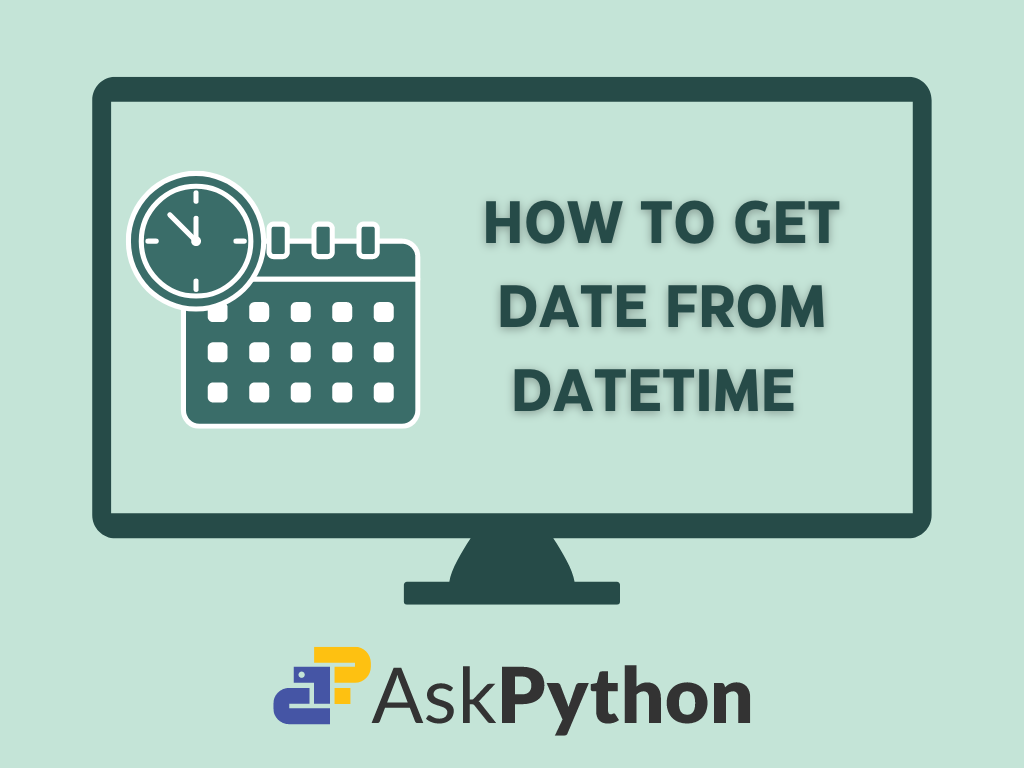# How to Convert a Datetime to DateWe know that datetime objects represent both the date and the time. On the other hand, the date object only represents the date. Say we have a datetime object but we are only concerned with the date data. We will need to convert the datetime object to a date object.

## Working with Dates and Times in Python

Python does not require distinct data types for dates and times. Instead, the Datetime module provides classes enabling us to manipulate date and time data. This built-in module negates the need for external installation.

The Datetime module comprises various classes, including date, time, datetime, and time delta, each providing distinct functions for handling dates and times. This article will focus solely on the date and datetime classes.

### Date Class

We use dateclass to create date objects. The dateclass has the following attributes:

• year (Eg: 2023)
• month (Any value between 1-12)
• day (Any valid date in the month that has been chosen)

An object of this class represents the date in the following format: YYYY-MM-DD.

The syntax of the constructor after importing the date class from datetime is:

```date(YYYY, MM, DD)
```

The constructor has three mandatory arguments: year, month, and day. All hold integer values.

Let’s create a date object.

```from datetime import date

#initialize constructor
d=date(2023, 3, 18)

print("The date is ", d)
```

#### OUTPUT:

```The date is 2023-03-18
```

### Datetime Class

We use datetime class to create datetime objects. We can work with both dates and times in datetime class.

Some of datetime’s attributes are as follows:

• year
• month
• date
• hour (an integer from 0-23)
• minute (an integer from 0-59)
• second (an integer from 0-59)
• microsecond (an integer from 0-999999)

The syntax of a constructor after importing datetime class from datetime module is as follows:

```class datetime(year, month, day, hour=0, minute=0, second=0, microsecond=0, tzinfo=None)
```

Here, only ‘year’, ‘month’, and ‘day’ arguments are mandatory. The rest of the arguments assume the default values if omitted in the code.

Let’s create a datetime object of class datetime.

```#creating a datetime object

from datetime import datetime

#initilize constructor
dt=(2023, 3, 18, 10, 10, 10, 100000)
print("The date and time is : ", dt)

#initilize constructor with only date parameters
dt=(2023, 3, 18)
print("With only initializing date parameters : ",dt)

```

#### OUTPUT:

```The date and time is 2023-03-18 10:10:10.100000
With only initializing date parameters : 2023:03:18 00:00:00

```

If you are puzzled by the datetime module, here’s an article that explores the datetime module in depth.

## Datetime to Date using date()

Up to this point, we have examined the datetime module and some of its classes, like “datetime” and “date.” Let’s finally understand how to convert a datetime object into a date object.

The date() method of the datetime class is the simplest way to obtain a date object. This method accepts a datetime object as input and returns a date object after extracting the date information from the object.

It will be clear once we look at an example.

```from datetime import datetime

# create a datetime object
datetime_obj =datetime(2023, 3, 20, 12, 30, 0)

# convert the datetime to date object
date_obj = datetime_obj.date()

print("The date object is : ", date_obj)
```

#### OUTPUT:

```The date object is :  2023-03-20
```

In the above code, we first create a datetime object, ‘datetime_obj’, with both date and time values. We then call the ‘date()’ method on ‘datetime_obj’. ‘Date()’ returns a new date object which we assign to ‘date_obj’. We then print the results.

## Strftime() and strptime()

We can convert a datetime object to a date object by first converting the datetime object to a string and then extracting the date components from the string. We use ‘strftime()’ method to convert the datetime object to a string.

To convert the string into a datetime object, we use ‘strptime()’, and after that, we convert it into a date object using date() method.

Let’s see how it works.

```from datetime import datetime

datetime_obj =datetime.now()

# format datetime object as string
datetime_str = datetime_obj.strftime('%Y-%m-%d')

# convert string to date object
date_obj =datetime.strptime(datetime_str, '%Y-%m-%d').date()

print(date_obj)
```

### Output generated is:

```2023-03-27

```